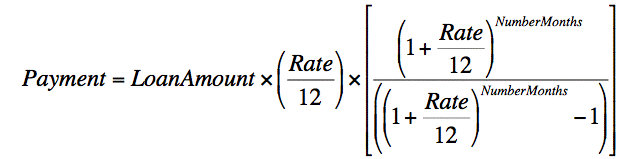# Problem with a math code

I have a math code that is giving to me the wrong answer, I check already the order of my code but I cannot see the problem, there is my code

\$loan = \$_POST[‘t_loan’];
\$rate = \$_POST[‘rate’];
\$monts = \$_POST[‘months’];
\$f_rate = \$rate/100;

\$payment = (\$loan * (\$f_rate / 12)) * (pow((1 + (\$f_rate / 12)), \$monts) / ((pow((1 + (\$f_rate / 12)), \$monts)) + 1));

\$payment = round(\$payment);
Print “\$payment”;

there is the equationWith a Loan of 18000 rate of 4.9(0.049) and 60 months to pay the result should be a 338 monthly payment but I am getting 41

I really appreciate any help

At the end of your line you have + 1, while on picture it says - 1

Yea I figure out early and changed but any way still cannot have the correct result, I changed the code again this one is the new one:

\$loan = \$_POST[‘t_loan’];
\$rate = \$_POST[‘rate’];
\$monts = \$_POST[‘months’];
\$f_rate = \$rate/100;

\$payment = \$loan * (\$f_rate / 12) * (pow((1 + (\$f_rate / 12)), \$monts) / pow((1 + (\$f_rate / 12)), \$monts) - 1);

\$payment = round(\$payment);
Print “\$payment”;

Hi there,

Try this line:
[php]
\$payment = \$loan * (\$f_rate / 12) * (pow((1 + (\$f_rate / 12)), \$monts) / (pow((1 + (\$f_rate / 12)), \$monts) - 1));
[/php]

in place of:
[php]
\$payment = \$loan * (\$f_rate / 12) * (pow((1 + (\$f_rate / 12)), \$monts) / pow((1 + (\$f_rate / 12)), \$monts) - 1);
[/php]

Let me know how it goes.

Thank you very much, now is working.

A++++